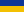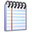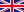On Pointwise Estimates for Convex Polynomial Approximation of Function with Fractional Derivatives of Arbitrary Order r > 4, r ie R / Petrova, T. O., Petrova, I. L. (2017)web address of the page http://jnas.nbuv.gov.ua/article/UJRN-0000862619  Mathematical and computer modelling. Series: Physical and mathematical sciences   Б - 2020  /Issue (2017, Вип. 16)Петрова Т. О., Петрова І. Л.Про поточкові інтерполяційні оцінки опуклого наближення функцій, що мають дробову похідну довільного порядку r > 4, r є R Бібліографічний опис:Петрова Т. О., Петрова І. Л. Про поточкові інтерполяційні оцінки опуклого наближення функцій, що мають дробову похідну довільного порядку r > 4, r є R. Математичне та комп'ютерне моделювання. Серія : Фізико-математичні науки. 2017. Вип. 16. С. 145-150. URL: http://jnas.nbuv.gov.ua/article/UJRN-0000862619Mathematical and computer modelling. Series: Physical and mathematical sciences    /Issue (2017, 16)Petrova T. O., Petrova I. L.On Pointwise Estimates for Convex Polynomial Approximation of Function with Fractional Derivatives of Arbitrary Order r > 4, r ie RCite:Petrova, T. O., Petrova, I. L. (2017). On Pointwise Estimates for Convex Polynomial Approximation of Function with Fractional Derivatives of Arbitrary Order r > 4, r ie R. Mathematical and computer modelling. Series: Physical and mathematical sciences , 16, 145-150. http://jnas.nbuv.gov.ua/article/UJRN-0000862619 [In Ukrainian].

 National Academy of Sciences of UkraineLibrary portal of National Academy of Sciences of Ukraine | LibNAShttp://libnas.nbuv.gov.uaVernadsky National Library of Ukraine (VNLU)Institute of Information Technologies of VNLU+38 (044) 525-36-24libnas@nbuv.gov.uaUkraine, 03039, Kyiv, Holosiivskyi Ave, 3, room 209